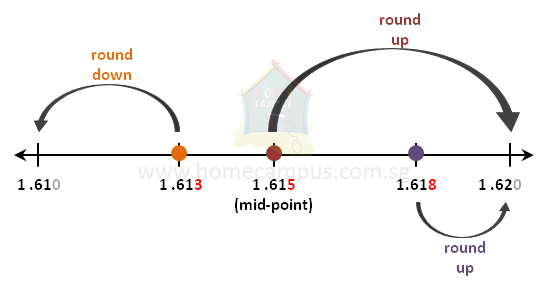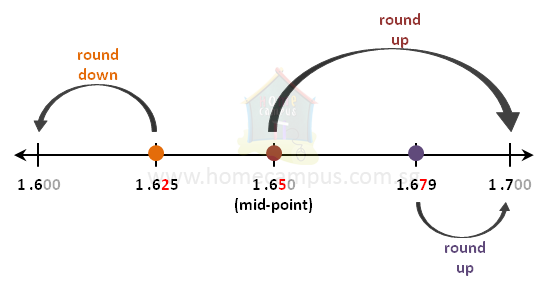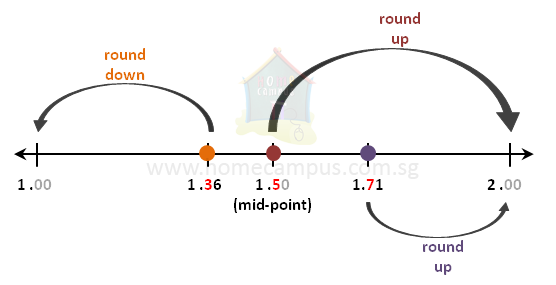## What is Rounding Off and How to Round Off Decimal Numbers?

Practice Unlimited Questions

#### What is rounding off?

Rounding off is a method to approximate values.

You round off a decimal number when:
1. It has too many decimal places and they do not make a difference to the desired value of the decimal number.
2. There is an endless series of decimal places:
Example:
1.    4  ÷  7  =  0.571428571428571428571428...
2.    1  ÷  6  =  0.16666666666666666666...
In such cases, you round off numbers to say that you only care about digits till a few decimal places (like tenths place, hundredths place, thousandths place, etc.) and that you do not care about the rest of the digits.

#### 1.  a)   Round off 1.615 to the hundredths place b)   Round off 1.613 to the hundredths place c)   Round off 1.618 to the hundredths place

Rounding off to the hundredths place (2 decimal places)

When rounding off to the hundredths place, you only care about the part of the number up to the hundredths place (second place after the decimal point). So,
1. Check the digit in the thousandths place (third place after the decimal point).
1. If it is less than 5, keep the digit in the hundredths place unchanged (i.e. round down)
2. If it is equal to or more than 5, add 1 to the digit in the hundredths place (i.e. round up)
2. Drop all the digits in the number after the hundredths place.1. 1.615 ≈ 1.62 (when rounded off to the hundredths place, it is rounded up because it is closer to 1.62 than to 1.61)
2. 1.613 ≈ 1.61 (when rounded off to the hundredths place, it is rounded down because it is closer to 1.61 than to 1.62)
3. 1.618 ≈ 1.62 (when rounded off to the hundredths place, it is rounded up because it is closer to 1.62 than to 1.61)

Notes:
1. All numbers to the left of the midpoint on the number line will be rounded down.
2. All numbers to the right of the midpoint including the midpoint itself will be rounded up.

#### 2. a)   Round off 1.625 to the tenths place b)   Round off 1.650 to the tenths place c)   Round off 1.679 to the tenths place

Rounding off to the tenths place (1 decimal place)

When rounding off to the tenths place, you only care about the part of the number up to the tenths place (first place after the decimal point). So,
1. Check the digit in the hundredths place (second place after the decimal point).
1. If it is less than 5, keep the digit in the tenths place unchanged (i.e. round down)
2. If it is equal to or more than 5, add 1 to the digit in the tenths place (i.e. round up)
2. Drop all the digits in the number after the tenths place.1. 1.625 ≈ 1.6 (when rounded off to the tenths place, it is rounded up because it is closer to 1.6 than to 1.7)
2. 1.650 ≈ 1.7 (when rounded off to the tenths place, it is rounded down because it is closer to 1.7 than to 1.6)
3. 1.679 ≈ 1.7 (when rounded off to the tenths place, it is rounded up because it is closer to 1.7 than to 1.6)

#### 3. a)   Round off 1.71 to the ones place b)   Round off 1.36 to the ones place c)   Round off 1.50 to the ones place

Rounding off to the ones place (nearest whole number)

When rounding off to the ones place, you only care about the part of the number up to the ones place, that is, you do not care about the decimal part of the number. So,
1. Check the digit in the tenths place (first place after the decimal point).
1. If it is less than 5, keep the digit in the ones place unchanged (i.e. round down)
2. If it is equal to or more than 5, add 1 to the digit in the ones place (i.e. round up)
2. Drop all the digits in the number after the ones place.1. 1.71 ≈ 2 (when rounded off to the ones place, it is rounded up because it is closer to 2 than to 1)
2. 1.36 ≈ 1 (when rounded off to the ones place, it is rounded down because it is closer to 1 than to 2)
3. 1.50 ≈ 2 (when rounded off to the ones place, it is rounded up because it is closer to 2 than to 1)